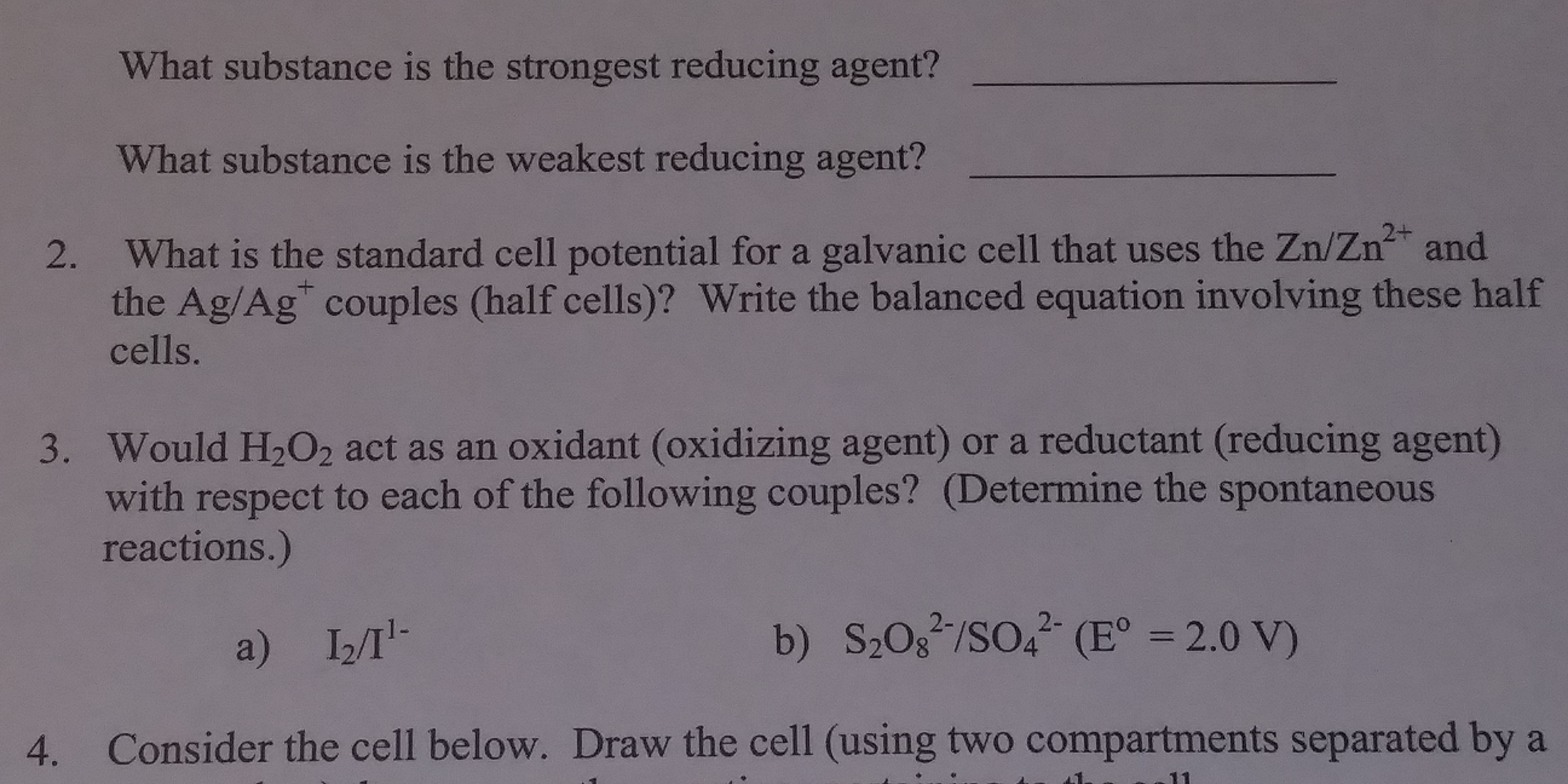# What substance is the strongest reducing agent?What substance is the weakest reducing agent?What is the standard cell potential for a galvanic cell that uses the Zn/Zn2+ andthe Ag/Ag" couples (half cells)? Write the balanced equation involving these halfcells.2.3.Would H202 act as an oxidant (oxidizing agent) or a reductant (reducing agent)with respect to each of the following couples? (Determine the spontaneousreactions.)a) I2T4.Consider the cell below. Draw the cell (using two compartments separated by a

Question
3 views

Number 2 and 3help_outlineImage TranscriptioncloseWhat substance is the strongest reducing agent? What substance is the weakest reducing agent? What is the standard cell potential for a galvanic cell that uses the Zn/Zn2+ and the Ag/Ag" couples (half cells)? Write the balanced equation involving these half cells. 2. 3. Would H202 act as an oxidant (oxidizing agent) or a reductant (reducing agent) with respect to each of the following couples? (Determine the spontaneous reactions.) a) I2T 4. Consider the cell below. Draw the cell (using two compartments separated by a fullscreen
check_circle

Step 1

The standard reduction potentials of the half electrodes ( from a standard data table) is :

Zn2+/Zn = -0.76 V

Ag+/Ag = 0.80 V

Step 2

The above values shows that Ag+ has a higher tendency of reduction than Zn2+.

Thus, silver electrode serves as cathode, since reduction takes place at cathode and zinc electrode serves as anode.

Step 3

The standard value of cell potent...

### Want to see the full answer?

See Solution

#### Want to see this answer and more?

Solutions are written by subject experts who are available 24/7. Questions are typically answered within 1 hour.*

See Solution
*Response times may vary by subject and question.
Tagged in

### Electrochemistry